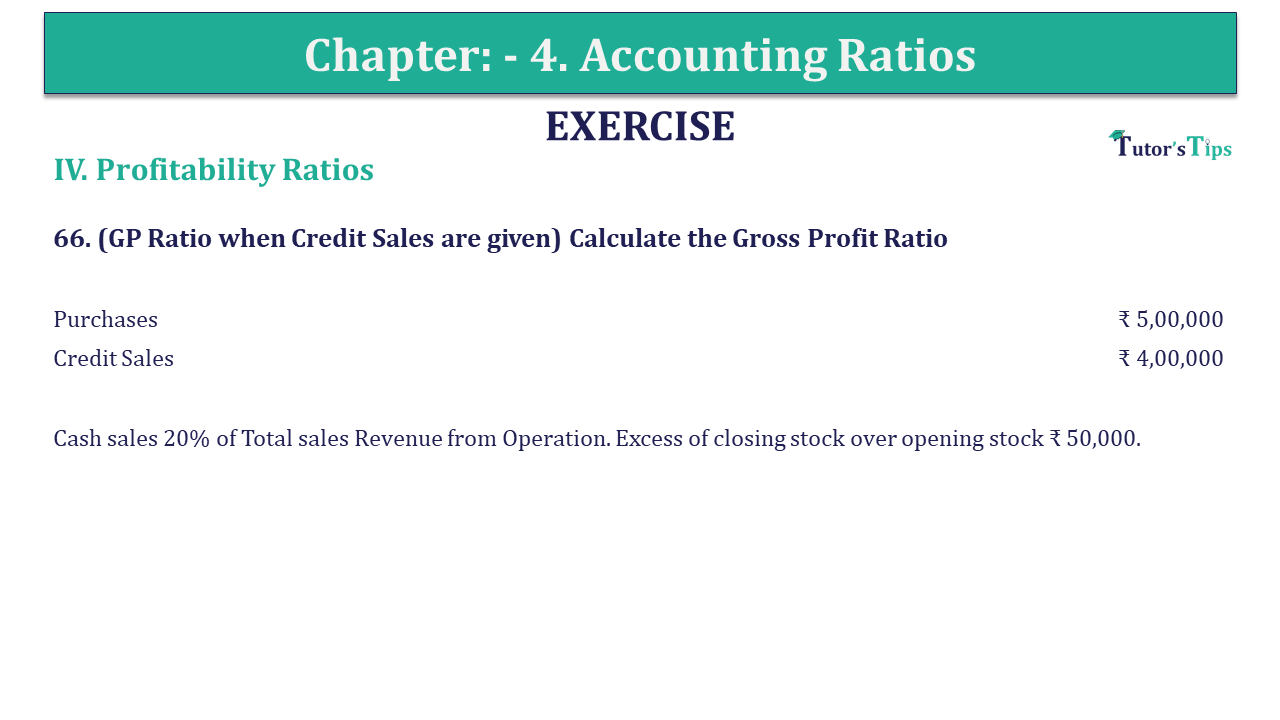# Question 66 Chapter 4 of +2-B – USHA Publication 12 ClassQ-66- CH-4 Book 2 - Usha Pub. +2 Book 2020 - Solution

Question 66 Chapter 4 of +2-B

66. (GP Ratio when Credit Sales are given) Calculate the Gross Profit Ratio

 Purchases ₹ 5,00,000 Credit Sales ₹ 4,00,000

Cash sales 20% of Total sales Revenue from Operation. Excess of closing stock over opening stock ₹ 50,000.

## The solution of Question 66 Chapter 4 of +2-B: –

Calculation of Gross Profit Ratio

 Total Revenue from Operation = Credit Revenue from Operation + Cash Revenue from Operations Let the Total Revenue from Operations = x Cash Revenue from Operations = 20% of x Credit Revenue from Operations = ₹ 4,00,000
 x = x X ₹ 4,00,000 5 5x = x + ₹ 20,00,000 5x-x = ₹ 20,00,000 x = ₹ 20,00,000 4 Total Sales = ₹ 5,00,000 Cash Revenue from Operations = 20 X ₹ 5,00,000 100 = ₹ 1,00,000

 Cost of goods sold = Purchases – Excess of Closing inventory over opening inventory = ₹ 5,00,000 – ₹ 50,000 = ₹ 4,50,000 Gross Profit = Total Sales – Cost of goods sold = ₹ 5,00,000 – ₹ 4,50,000 Purchase = ₹ 50,000
 Gross Profit Ratio = Gross Profit X 100 Sales
 = ₹ 50,000 X ₹ 1,50,000 ₹ 5,00,000 = 10%

Also, Check out the solved question of previous Chapters: –